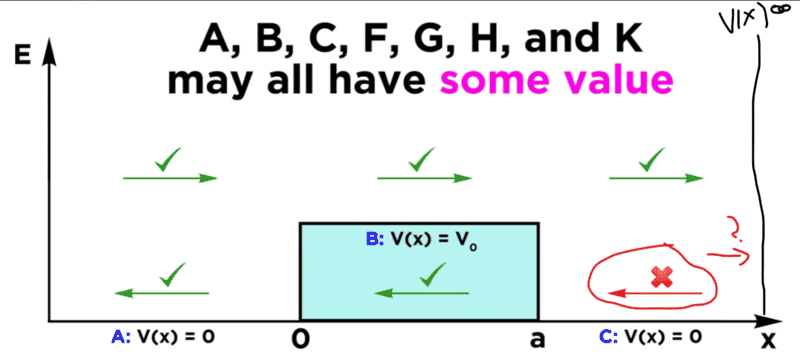# Quantum Tunneling to an infinite wall

maguss182
Homework Statement:
Need to find what the boundary condition is for an Energy hitting an infinity wall of potential
Relevant Equations:
Ce^ikx+De-ikx - Wavefunction for 3rd region I need to apply boundary conditions to.
I have the equations for all three regions but usually for region 3, which is Ce^ikx+De-ikx, the C term would be zero since there is no reflection, but with the infinite wall would it reflect? Would the whole wavefunction go to zero like when working with the infinite square wall? I'm stuck on this part, any help would be greatly appreciated

I edited this screenshot I took from a youtube video, to make it clearer what I'm referring tooHomework Helper
Hello,

You want to read your post and clarify a lot of things. I don't see a region 3: do I have to guess ? If so, C is a good candidate. But then another C than the one in ##C e^{ikx} + D e^{-ikx}##, I suppose.

What about ##x<0##? Another solid wall with ##V=\infty## where the y (E?) axis is drawn? So we have a particle in a box with a barrier? Or a region extending to ##-\infty## meaning we have particles coming from the left to find a barrier and after that our wall ?
I have the equations
Why not share them ? And your solutions as well --- if only to provide some clarity !

##\ ##

maguss182
Sorry I'll try to clarify a bit more, you have a particle coming in from the left, it hits a barrier of potential v0, then it continues and hits an infinite wall at some point x, my question is what happens to the wave function at that point x when it hits the infinite wall, does it go to zero like in an infinite square well, or does it have a reflection? I marked the regions, and the red line is the infinite wall that the particle heads towards.

My equations are for
Region 1= Aeikx+Be-ikx
Region 2 = Fek0x + Ge-k0x
Region 3 = Ceikx+De-ik0x

If the infinite wall (red on the picture) wasn't there, then C would be zero since there is no reflection, but you still have D, however with the infinite wall there, does it all go to zero? Or is there a reflection C?

I hope that's a little more clear, thank you!

#### Attachments

Staff Emeritus
Homework Helper
First, keep in mind that the wave function should be defined for all ##x##. In particular, what's the value of ##\psi## in the region where the potential is infinite?

Second, the wave function needs to be continuous. What does that tell you about how ##\psi## behaves as you approach the wall from the left in region 3? What does that imply about how ##C## and ##D## are related?

Last edited:
maguss182
So in my example, x=o when ##\psi## which would make e^0=1 so my region 3 equation becomes C+D=0 or C=D?

Homework Helper
2022 Award
does it go to zero like in an infinite square well, or does it have a reflection?
This statement does not make sense.
The wavefunction you are trying to write down is an eigenstate that exists for all time. The various pieces of it represent incoming and outgoing waves at infinity. The boundary condition that the amplitude must vanish at the "wall" will require that the left and right fluxes match. The presence of that region will allow for "resonant" states to exist. There are boundary conditions at each of the three boundaries sufficient to solve for all of the constants.
So in my example, x=o when ψ which would make e^0=1 so my region 3 equation becomes C+D=0 or C=D?

If the wall is at position x=b then that will generate contions on C and D that will involve ##\Psi (b)##
I am not sure I understand which region is which from your pictures

Staff Emeritus
Homework Helper